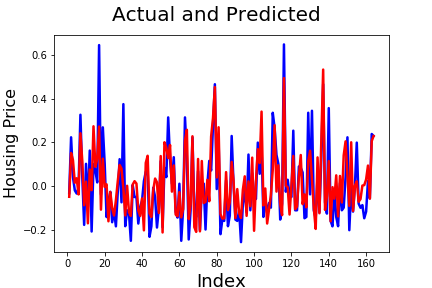# Case Study-2-House Sale Price Prediction

## Linear regression Case Study : House Sale Price Prediction

In this blog, you will find one more example of a Linear regression case study and this case study is about optimizing the sale prices of the properties based on important factors such as area, bedrooms, parking, etc.

#### Step1: Importing and Understanding Data.

```
import pandas as pd
import numpy as np

# Importing Housing.csv

# Looking at the first five rows

Output:

 price area bedrooms bathrooms stories mainroad guestroom basement hotwaterheating airconditioning parking prefarea furnishingstatus 13300000 7420 4 2 3 yes no no no yes 2 yes furnished 12250000 8960 4 4 4 yes no no no yes 3 no furnished 12250000 9960 3 2 2 yes no yes no no 2 yes semi-furnished 12215000 7500 4 2 2 yes no yes no yes 3 yes furnished 11410000 7420 4 1 2 yes yes yes no yes 2 no furnished

#### Step2: Data Preparation.

``` # Converting Yes to 1 and No to 0
housing['guestroom'] = housing['guestroom'].map({'yes': 1, 'no': 0})
housing['basement'] = housing['basement'].map({'yes': 1, 'no': 0})
housing['hotwaterheating'] = housing['hotwaterheating'].map({'yes': 1,'no': 0})
housing['airconditioning'] = housing['airconditioning'].map({'yes': 1,'no': 0})
housing['prefarea'] = housing['prefarea'].map({'yes': 1, 'no': 0})
```

#### Step3: Creating dummy variables for variable ‘furnishingstatus’ and dropping the first one.

` status = pd.get_dummies(housing['furnishingstatus'],drop_first = True)`

#### Step4: Adding the results to the master dataframe.

`housing = pd.concat([housing,status],axis=1)`

#### Step5: Dropping the variable ‘furnishingstatus’.

` housing.drop(['furnishingstatus'],axis=1,inplace=True)`

#### Step6: Create new variables.

```
# Let us create the new metric and assign it to "areaperbedroom"
housing['areaperbedroom'] = housing['area']/housing['bedrooms']
# Metric: bathrooms per bedroom
housing['bbratio'] = housing['bathrooms']/housing['bedrooms']
```

#### Step7: Data normalization. (Rescaling features)

``` #defining a normalisation function
def normalize (x):
return ( (x-np.mean(x))/ (max(x) - min(x)))

# applying normalize ( ) to all columns
housing = housing.apply(normalize)
```

#### Step8: Split data into training and testing sets.

``` # Putting feature variable to X
X = housing[['area', 'bedrooms', 'bathrooms', 'stories', 'mainroad',
'guestroom', 'basement', 'hotwaterheating', 'airconditioning',
'parking', 'prefarea', 'semi-furnished', 'unfurnished',
'areaperbedroom', 'bbratio']]

# Putting response variable to y
y = housing['price']
```

#### Step9: Split X and y into X_train, X_test, y_train,y_test.

```
from sklearn.cross_validation import train_test_split
X_train, X_test, y_train, y_test = train_test_split(X, y, train_size=0.7 ,test_size = 0.3, random_state = 100)
```

#### Step10: Use the VIF metric to remove unnecessary variables. Details of variance inflation factor can be found here.

```
# UDF for calculating vif value
def vif_cal(input_data, dependent_col):
vif_df = pd.DataFrame( columns = ['Var', 'Vif'])
x_vars=input_data.drop([dependent_col], axis=1)
xvar_names=x_vars.columns
for i in range(0,xvar_names.shape):
y=x_vars[xvar_names[i]]
x=x_vars[xvar_names.drop(xvar_names[i])]
rsq=sm.OLS(y,x).fit().rsquared
vif=round(1/(1-rsq),2)
vif_df.loc[i] = [xvar_names[i], vif]
return vif_df.sort_values(by = 'Vif', axis=0, ascending=False, inplace=False)
```

#### Step11: Use RFE to reduce variables.

For this Linear regression case study, we will use ‘Scikit’ learn library.

```
from sklearn.feature_selection import RFE
from sklearn.linear_model import LinearRegression
```

#### Step12: RFE with the output number of the variable equal to 9.

```
lm = LinearRegression()
rfe = RFE(lm, 9) # running RFE
rfe = rfe.fit(X_train, y_train)
print(rfe.support_) # Printing the boolean results
print(rfe.ranking_)
```

Output:

``````[ True False  True  True  True False False  True  True False  True False
False  True  True]
[1 3 1 1 1 4 6 1 1 2 1 7 5 1 1``````

#### Step13: Get all variables.

```
col = X_train.columns[rfe.support_]
X_train_rfe = X_train[col]
```

#### Step14: Building a model using sklearn.

```
import statsmodels.api as sm
lm = sm.OLS(y_train,X_train_rfe).fit() # Run the model
```

#### Step15: Let’s see the summary of our linear model.

` print(lm.summary())`

Output:

```                           OLS Regression Results
==============================================================================
Dep. Variable:                  price   R-squared:                       0.660
Method:                 Least Squares   F-statistic:                     80.14
Date:                Mon, 13 Apr 2020   Prob (F-statistic):           1.88e-81
Time:                        15:56:09   Log-Likelihood:                 369.54
No. Observations:                 381   AIC:                            -719.1
Df Residuals:                     371   BIC:                            -679.7
Df Model:                           9
Covariance Type:            nonrobust
===================================================================================
coef    std err          t      P&amp;amp;gt;|t|      [0.025      0.975]
-----------------------------------------------------------------------------------
const               0.0034      0.005      0.704      0.482      -0.006       0.013
area                0.7022      0.130      5.421      0.000       0.447       0.957
bathrooms           0.1718      0.098      1.759      0.079      -0.020       0.364
stories             0.0814      0.019      4.321      0.000       0.044       0.118
mainroad            0.0647      0.014      4.470      0.000       0.036       0.093
hotwaterheating     0.1002      0.022      4.523      0.000       0.057       0.144
airconditioning     0.0776      0.011      6.806      0.000       0.055       0.100
prefarea            0.0631      0.012      5.286      0.000       0.040       0.087
areaperbedroom     -0.4095      0.143     -2.868      0.004      -0.690      -0.129
bbratio             0.1156      0.080      1.450      0.148      -0.041       0.272
==============================================================================
Omnibus:                       85.512   Durbin-Watson:                   2.108
Prob(Omnibus):                  0.000   Jarque-Bera (JB):              273.429
Skew:                           0.998   Prob(JB):                     4.22e-60
Kurtosis:                       6.638   Cond. No.                         46.6
==============================================================================```

#### Step16: Calculate the Vif value for all variables and verify the values of each variable. If the value is more, you can remove the variable from the model and re-run the model.

` vif_cal(input_data=housing.drop(['area','bedrooms','stories','basement','semi-furnished','areaperbedroom'], axis=1), dependent_col="price")`

Output:

`````` 	Var 	Vif
0 	bathrooms 	2.35
8 	bbratio 	2.19
5 	parking 	1.12
4 	airconditioning 	1.11
6 	prefarea 	1.09
7 	unfurnished 	1.07
2 	guestroom 	1.06
3 	hotwaterheating 	1.04``````

#### Step17: At this point, VIF and P-values look good for the model, we can proceed to test our model on test data.

```
X_test_rfe = X_test[col]
# Making predictions
y_pred = lm.predict(X_test_rfe)
```

#### Step18: Evaluate the model, which we built for the linear regression case study.

```
# Importing the required libraries for plots.
import matplotlib.pyplot as plt
import seaborn as sns
%matplotlib inline

c = [i for i in range(1,165,1)] # generating index
fig = plt.figure()
plt.plot(c,y_test, color="blue", linewidth=2.5, linestyle="-") #Plotting Actual
plt.plot(c,y_pred, color="red",  linewidth=2.5, linestyle="-") #Plotting predicted
fig.suptitle('Actual and Predicted', fontsize=20)              # Plot heading
plt.xlabel('Index', fontsize=18)                               # X-label
plt.ylabel('Housing Price', fontsize=16)                       # Y-label
```

Output:

####Step19: Verify Root Mean Square Error of our model.

``` import numpy as np
from sklearn import metrics
print('RMSE :', np.sqrt(metrics.mean_squared_error(y_test, y_pred)))```

Output:

``RMSE : 0.108203525381``

Conclusion

The above Linear regression case study is about predicting sale price of a house. Please follow this for more insights about Linear Regression.